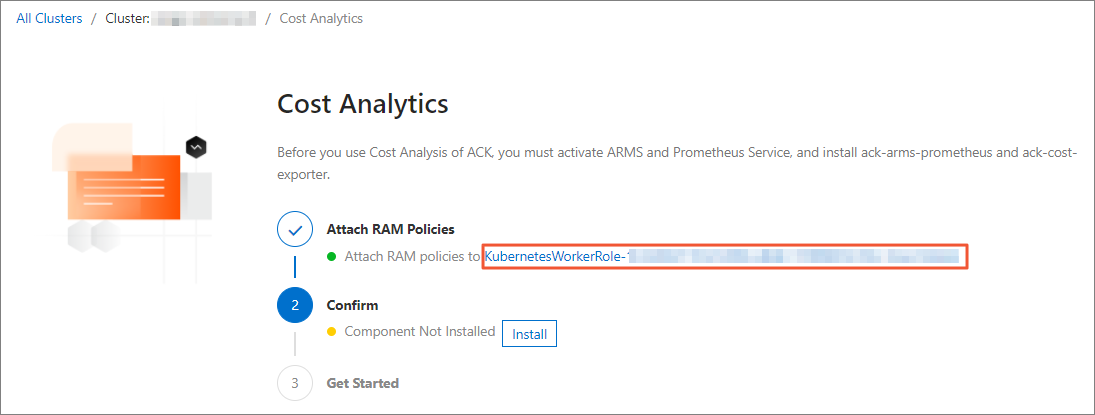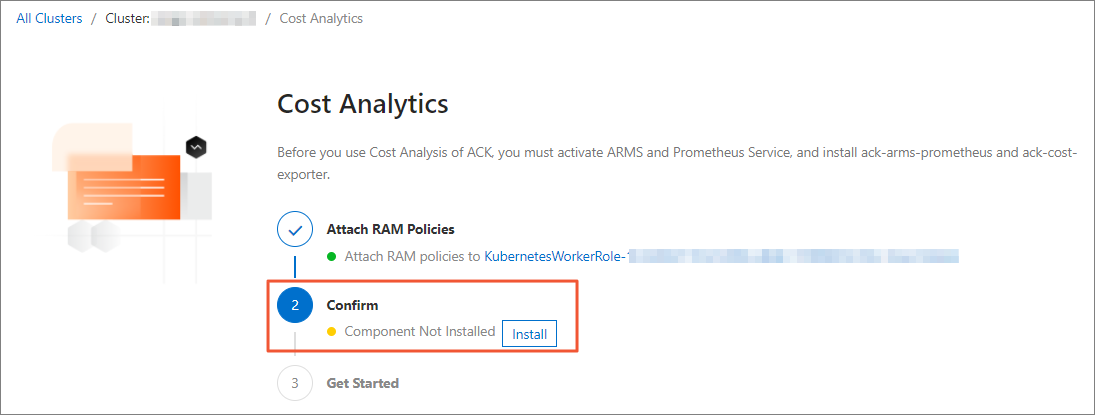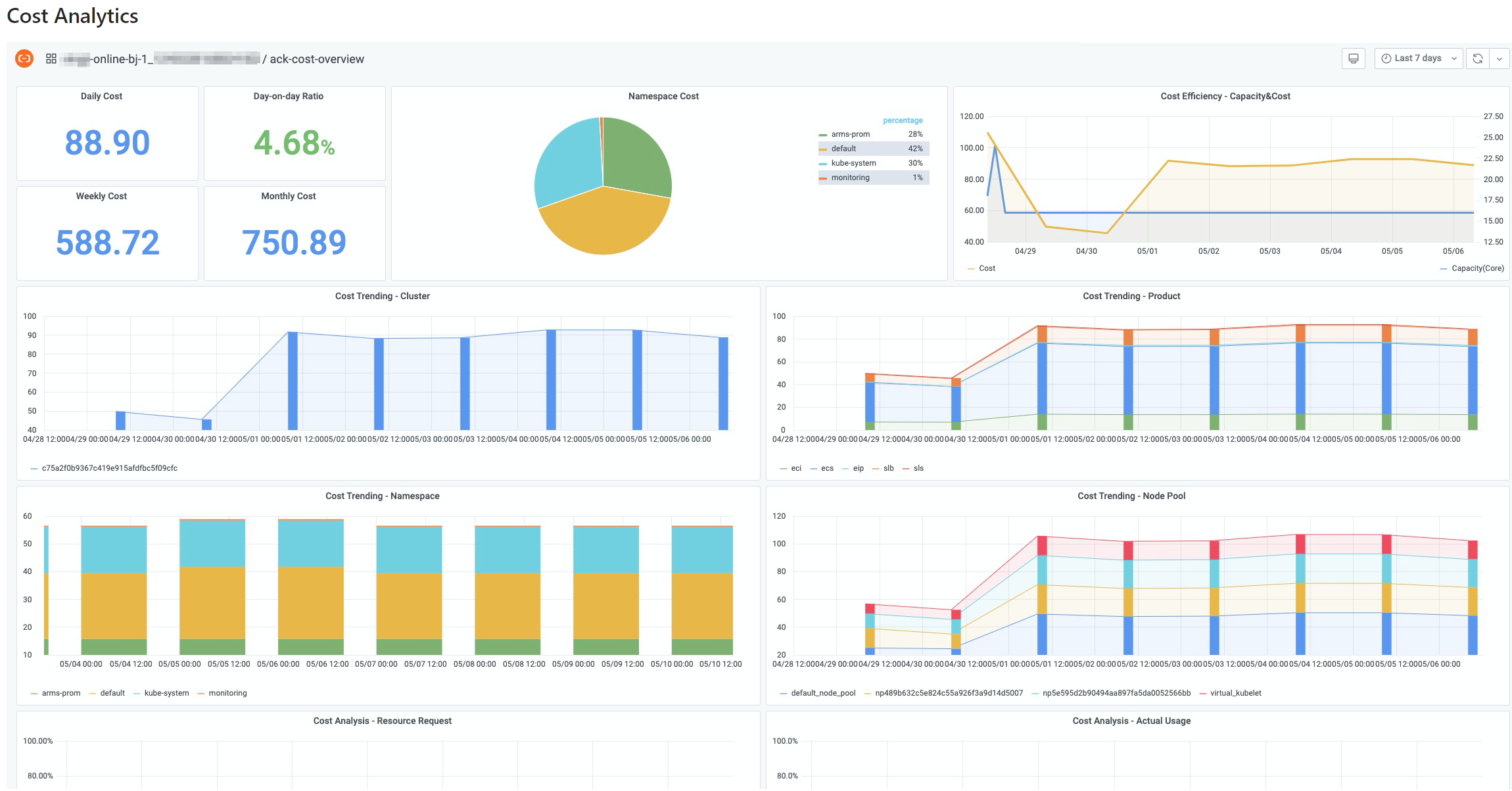The cost analysis feature of Container Service for Kubernetes (ACK) provides analysis on resource usage and cost distribution. This provides suggestions for enterprise IT administrators on how to improve resource utilization and reduce the cost. This topic introduces the cost analysis feature and how to configure cost analysis.

## Features

The following features are provided:
• Cost analysis of cloud resources

Aggregates the costs of all cloud resources within a Kubernetes cluster. Compares the cost of the previous day with that of the same date of the previous year. Compares the cost of the previous day with that of the day before the previous day.

• Cost trend analysis

Analyzes the cost trends of all cloud resources. Lists cloud resources with the highest cost contributions. This provides information that helps you reduce costs.

• Suggestions on cost saving

Analyzes the cost trend of a Kubernetes cluster. Provides correlation analysis between resource usage and cost contribution. Provides suggestions to reduce costs.

• Real-time cost forecast

Simulates cost calculation based on the real-time prices of all computing resources within a cluster. Provides hourly cost forecasts. Multiple billing methods, such as subscription, pay-as-you-go, and preemptible instances, are supported.

• Cost allocation based on namespaces

Allocates the cost of a cluster based on namespaces. Provides the real-time cost forecast, cost trend, and cost contribution of each namespace.

• Optimization of application costs

Lists the top 10 applications with the highest cost contributions. Provides the cost trends of the top 10 applications. This provides information that helps you improve the cost-effectiveness of an application.

## Configure cost analysis

1. Log on to the ACK console.
2. In the left-side navigation pane of the ACK console, click Clusters.
3. On the Clusters page, find the cluster that you want to manage and click the name of the cluster or click Details in the Actions column. The details page of the cluster appears.
4. In the left-side navigation pane of the details page, choose Operations > Cost Analysis.
5. On the Cost Analysis page, click the worker Resource Access Management (RAM) role KubernetesWorkerRole-*** of the cluster to go to the RAM console and modify the permission policy.1. On the RAM Roles page, click the name of the K8sWorkerRolePolicy-*** policy.
2. On the Policies page, click Modify Policy Document.
3. In the Modify Policy Document panel, add the following content and then click OK.
``````        {
"Action": [
"bssapi:QueryInstanceBill"
],
"Resource": "*",
"Effect": "Allow"
},
{
"Action": [
"ecs:DescribeSpotPriceHistory",
"ecs:DescribeInstances",
"ecs:DescribePrice"
],
"Resource": "*",
"Effect": "Allow"
}``````
Note To specify multiple actions, add a comma (,) to the end of the content of each action before you enter the content of the next action.
6. On the Cost Analysis page, click Install.7. On the Cost Analysis page, you can view a dashboard that provides visualized information about the cost.
Note After cost analysis is enabled, the bills are displayed at 08:00:00 (UTC+8) on the next day.## Use cost analysisThe following table describes the features of the dashboard.

No. Feature Description
Analyze the resource waste of the cluster based on the cost trend This figure shows the details of the cost. Daily Cost indicates the cost of the previous day. Day-on-day Ratio indicates the growth rate of the cost of the previous day.

If the growth rate is in green color, it indicates that the cost of the previous day is reduced compared with the cost of the day before the previous day. If the growth rate is in red color, it indicates that the cost of the previous day is increased compared with the cost of the day before the previous day

The yellow curve indicates the resource consumption. The blue curve indicates the actual resource capacity of the cluster. In common cases, the two curves may be correlated with each other.

If the two curves represent different trends, it indicates changes to the average cost of one CPU core. In this case, you can check whether resources of higher prices are used.

The cost trend within a time period.
Allocate the cost based on namespaces

A cluster may contain nodes of different specifications and billing methods. When you allocate the cost based on namespaces, you must consider the price differences of nodes that host pods in different namespaces, not only the resource consumption of different namespaces.

The cost analysis feature converts the real-time cost of each node by using alibaba-cloud-price-exporter. The cost of a namespace is calculated based on the following formula:
``Σ (Pod resource requests/Node capacity) × Node unit price``

This provides a precise method to calculate the cost of a namespace. However, discounts, vouchers, and subscriptions may cause the actual cost to be different from the cost calculated based on this formula. To obtain the actual cost of a namespace, you can multiply the actual cost of the cluster by the ratio of the cost of this namespace to the total cost of all namespaces.

Analyze the costs of cloud resources based on cost trends A cluster may use multiple cloud resources. The costs of cloud resources vary based on the billing rules and how the cloud resources are used by the cluster. This feature provides the cost trends and contributions of different cloud resources. This provides information that helps you reduce the cost.

## FAQ

Cost analysis is based on the monitoring data and cost data. The collection of monitoring data starts within 3 minutes after you enable cost analysis. Cost data is displayed at 08:00:00 (UTC+8) on the next day.
The cost of a namespace is calculated based on cost estimation and is not directly related to the actual bills. Therefore, the cost estimation is based on the list prices. However, the actual cost of a cluster can be reduced by vouchers, discounts, and cost saving plans. As a result, the total estimated cost of all namespaces may not equal the actual cost of the cluster. To obtain the actual cost of a namespace, you can multiply the actual cost of the cluster by the ratio of the cost of this namespace to the total cost of all namespaces.